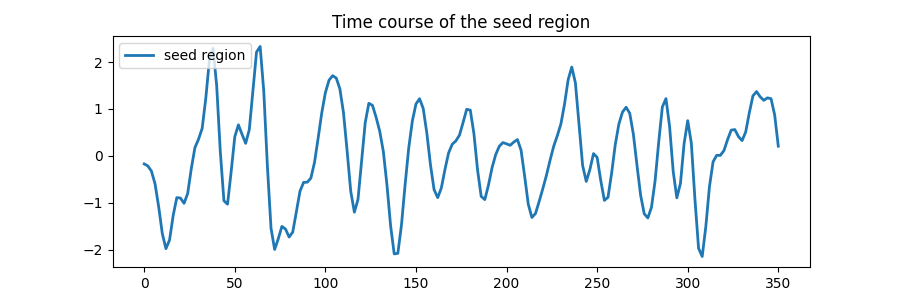# Default Mode Network extraction of ADHD dataset#

This example shows a full step-by-step workflow of fitting a GLM to data extracted from a seed on the Posterior Cingulate Cortex and saving the results.

More specifically:

1. A sequence of fMRI volumes are loaded.

2. A design matrix with the Posterior Cingulate Cortex seed is defined.

3. A GLM is applied to the dataset (effect/covariance, then contrast estimation).

4. The Default Mode Network is displayed.

Note

If you are using Nilearn with a version older than `0.9.0`, then you should either upgrade your version or import maskers from the `input_data` module instead of the `maskers` module.

That is, you should manually replace in the following example all occurrences of:

```from nilearn.maskers import NiftiMasker
```

with:

```from nilearn.input_data import NiftiMasker
```
```import numpy as np
from nilearn import datasets, plotting
from nilearn.glm.first_level import (
FirstLevelModel,
make_first_level_design_matrix,
)
```

## Prepare data and analysis parameters#

Prepare the data.

```adhd_dataset = datasets.fetch_adhd(n_subjects=1)

# Prepare timing
t_r = 2.0
slice_time_ref = 0.0
n_scans = 176

# Prepare seed
pcc_coords = (0, -53, 26)
```

## Estimate contrasts#

Specify the contrasts.

```seed_masker = NiftiSpheresMasker(
[pcc_coords],
detrend=True,
standardize="zscore_sample",
low_pass=0.1,
high_pass=0.01,
t_r=2.0,
memory="nilearn_cache",
memory_level=1,
verbose=0,
)
frametimes = np.linspace(0, (n_scans - 1) * t_r, n_scans)
design_matrix = make_first_level_design_matrix(
frametimes,
hrf_model="spm",
)
dmn_contrast = np.array( +  * (design_matrix.shape - 1))
contrasts = {"seed_based_glm": dmn_contrast}
```

## Perform first level analysis#

Setup and fit GLM.

```first_level_model = FirstLevelModel(t_r=t_r, slice_time_ref=slice_time_ref)
first_level_model = first_level_model.fit(
)
```
```/home/yasmin/nilearn/nilearn/nilearn/glm/first_level/first_level.py:75: UserWarning:

Mean values of 0 observed.The data have probably been centered.Scaling might not work as expected
```

Estimate the contrast.

```print("Contrast seed_based_glm computed.")
z_map = first_level_model.compute_contrast(
contrasts["seed_based_glm"], output_type="z_score"
)

# Saving snapshots of the contrasts
filename = "dmn_z_map.png"
display = plotting.plot_stat_map(
z_map, threshold=3.0, title="Seed based GLM", cut_coords=pcc_coords
)
marker_coords=[pcc_coords], marker_color="g", marker_size=300
)
display.savefig(filename)
print(f"Save z-map in '{filename}'.")
``````Contrast seed_based_glm computed.
Save z-map in 'dmn_z_map.png'.
```

## Generating a report#

It can be useful to quickly generate a portable, ready-to-view report with most of the pertinent information. This is easy to do if you have a fitted model and the list of contrasts, which we do here.

```from nilearn.reporting import make_glm_report

report = make_glm_report(
first_level_model,
contrasts=contrasts,
cluster_threshold=15,
min_distance=8.0,
plot_type="glass",
)
```

We have several ways to access the report:

```# report  # This report can be viewed in a notebook
# report.save_as_html('report.html')
# report.open_in_browser()
```

Total running time of the script: ( 0 minutes 23.480 seconds)

Estimated memory usage: 588 MB

Gallery generated by Sphinx-Gallery